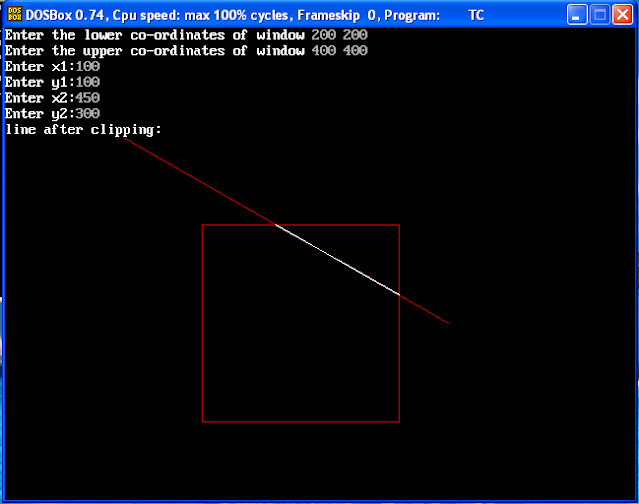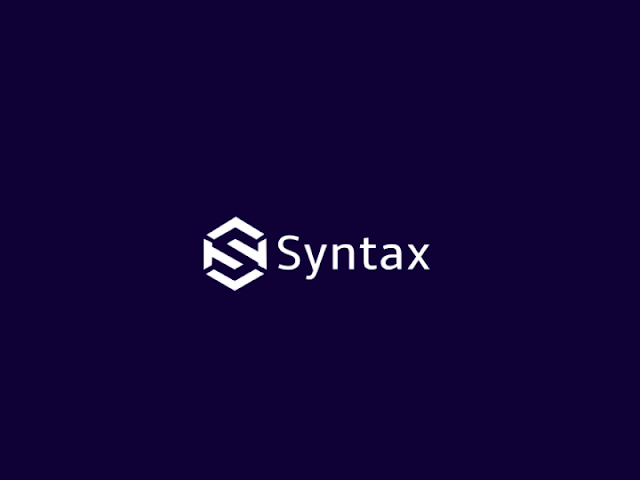# Liang Barsky AlgorithmImage Source - Google | Image By - pracspedia
• Parametric equation of line is given by,

x = x1 + (x2 - x1) * u

y = y1 + (y2 - y1) * u

Where, 0 <= u <= 1

x2 - x1 = delta x

y2 - y1 = delta y

Replacing this values in equation,

x = x1 + u * delta x

y = y1 + u * delta y

• The point clipping condition can be written in parametric form as,

xwmin <= x1 + delta x * u <= xwmax

ywmin <= y1 + delta y * u <= ywmax

• Each of this 4 inequality is represented as,

u * pk <= qk, k = 1, 2, 3, 4

Where,

p1 = - delta x, q1 = x1 - xwmin

p2 = delta x, q2 = xwmax - x1

p3 = - delta y, q3 = y1 - ywmin

p4 = delta y, q4 = ywmax - y1

• Any line i.e parallel to one of the clipping boundry has pk = 0.

• If for that value of k, we get qk < 0 then line is completely outside the window & can be eliminated if qk >= 0, the line is parallel to the window.

• When pk < 0 the line is from outside to inside if pk > 0, the line is from inside to outside for non - zero value of pk, we can calculate u = qk / pk.

• For each line we calculate u1 & u2,

The value of u1 is determinded for the line which proceeds from outside to inside p < 0, for this we calculate rk = qk / pk.

• The value of u1 is taken as the largest of the r value.

• Conversely, the value of u2 is determined for the line which proceeds from inside to outside (p > 0).

• Again Rk is calculated and u2 is the minimum of r value.

• If u1 > u2, the line is completely outside & rejected otherwise endpoints are calculated using parameter u.

• Initialize u1 & u2 to 0 and 1 respectively.

• For each boundry calculate p & q, if p < 0.

• R is used to update otherwise if p > 0, r is used to update u2 if updating u1 & u2 results in u1 > u2.

• We reject the line otherwise we update u.

• If p = 0 & q < 0, we discard the line since it is parallel & outside the window.

• The endpoints are calculated using parameter u1 & u2.

Clipline (x1, y1, x2, y2, xwmin, xwmax, ywmin, ywmax)

{

u1 = 0.0, u2 = 1.0

dx = x2 - x1 ,dy = y2 - y1

if (cliptest (-dx, x1 - xwmin, u1, u2))

{

if (cliptest (dx, xwmax - x1, u1, u2))

{

if (cliptest (-dy, y1 - ywmin, u1, u2))

{

if (cliptest (dy, ywmin - y1, u1, u2))

{

if (u2 < 1.0)

{

x2 = x1 + u2 * dx

y2 = y1 + u2 * dy

}

if (u1 > 0.0)

{

x1 = x1 + u1 * dx

y1 = y1 + u1 * dy

}

bresenham (round (x1), round (y1), round (x2), round (y2))

}

}

}

}

Cliptest (p, q, u1, u2)

{

retvalue = true

if (p < 0)

{

r = q / p

if (r > u2)

{

retvalue = false

else

if (r > u1)

u1 = r

}

else if (p > 0)

{

r = q / p

if (r < u1)

{

retvalue  = false

else if (r < u2)

u2  = r

}

else

{

if (q < 0)

retvalue  = false

}

return (retvalue);

}

2-D Rotation In Computer Graphics

Segments In Computer Graphics

Comment your views on this Article :)

## Thank you for visiting my blog :)

#### No comments

Comment your views on this article

### Syntax Explanation of System Software Programs